The Effect of Calculators in a Professional WorkplaceAs a Professional, be it in Mathematics, Engineering, Medicine, Physics or any other career path, there is indeed the need to use various tools or technology in your field to achieve high end results. Sometimes these technologies are seen to produce positive results. Other times, it seems to have adverse effects

When dealing with advanced Mathematical problems, or trying to prove mathematical theorems, lots of research work, mundane steps, and records of theses findings, must be put into consideration. For example a mathematician or physicist will have to understand some programming languages, like python, Matlab etc. to compute equations and generate graphical representation to back up proof of their findings, experiments or research work, in a means to describe in terms of numbers and figures for example, the rate at which an action occurs (like the force of gravity pulling a falling stone at 9.8m/sec^2). It might also be required as a guide in helping publish a professional work or findings to other professionals in the field.

Nickzom Calculator Converts Units of Typography

According to Wikipedia,

Typography is the art and technique of arranging type to make written language legiblereadable, and appealing when displayed. The arrangement of type involves selecting typefacespoint sizesline lengths, line-spacing (leading), and letter-spacing (tracking), and adjusting the space between pairs of letters (kerning). The term typography is also applied to the style, arrangement, and appearance of the letters, numbers, and symbols created by the process. Type design is a closely related craft, sometimes considered part of typography; most typographers do not design typefaces, and some type designers do not consider themselves typographers. Typography also may be used as a decorative device, unrelated to communication of information.

There are various units of typography. Nickzom Calculator converts the following units.

• Twip
• Centimeters
• Millimeters
• Character (X)
• Character (Y)
• Pixel (X)
• Pixel (Y)
• Inch
• Pica (Computer)
• Pica (Printer’s)
• PostScript Point
• Point (Computer)
• Point (Printer’s)
• En

As always the first thing to do is? Proceed to the Calculator Map. Scroll down to Switches and Click on Typography Switches.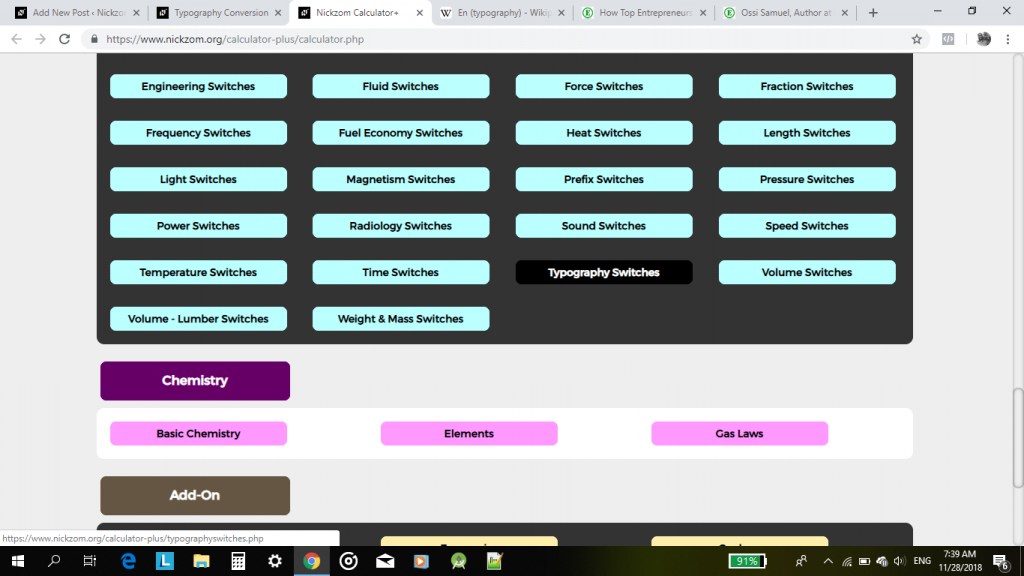Nickzom Calculator Solves for Lowest Common Multiples and Highest Common Factor in Pre Algebra

According to Wikipedia,

In arithmetic and number theory, the least common multiplelowest common multiple, or smallest common multiple of two integers a and b, usually denoted by LCM(ab), is the smallest positive integer that is divisible by both a and b.] Since division of integers by zero is undefined, this definition has meaning only if a and b are both different from zero. However, some authors define LCM (a,0) as 0 for all a, which is the result of taking the LCM to be the least upper bound in the lattice of divisibility.

The LCM is the “lowest common denominator” (LCD) that can be used before fractions can be added, subtracted or compared. The LCM of more than two integers is also well-defined: it is the smallest positive integer that is divisible by each of them.

According to Math is Fun,

The highest common factor or greatest common divisor is the highest number that divides exactly into two or more numbers. It is the “greatest” thing for simplifying fractions!

Nickzom Calculator is capable of computing for a small or large range of numbers in determining the lowest common multiple or highest common factor

When it comes to lowest common multiple, popularly known as LCM for a range of numbers, it takes the multiples of all the numbers that can divide the range of numbers till the range of numbers are all 1.

When it comes to the highest common factor, popularly known as HCF or GCD, it takes the multiple of the numbers that can only divide through all the ranges of numbers simultaneously.

If you have been following this blog by now, you must have known that the first thing to do is?

Proceed to the Calculator Map

Nickzom Calculator Calculates the Mean for Discrete and Continuous Data with Frequency under Statistics

Nickzom Calculator is capable of calculating a lot of problems on statistics and one of them is mean under measures of central tendency.

To this is very simple.

To access this functionalities in Nickzom Calculator, you need to purchase our professional version – Nickzom Calculator+ (The Calculator Encyclopedia). You can access this software via any of these channels:

Straight up go to Calculator Map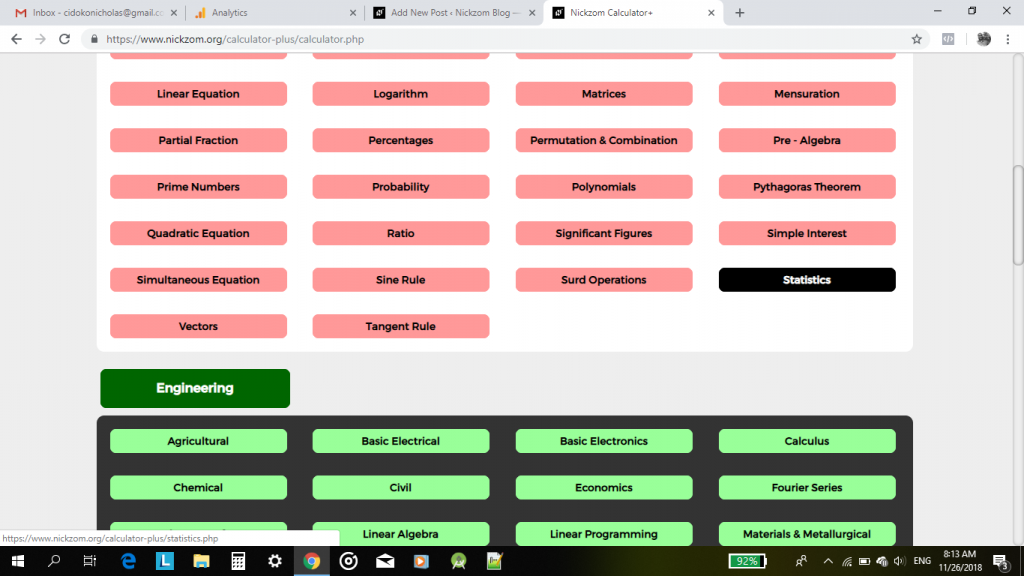Click on StatisticsBelow, is a video displaying Nickzom Calculator solving the mathematical mean  of discrete and continuous data with and without frequencies.

How to Get Answers of a 2 By 2 Matrix Linear Programming Maximization Problem Without Artificial Variables Using Nickzom Calculator

According to Google Dictionary,

Linear Programming is a mathematical technique for maximizing or minimizing a linear function of several variables, such as output or cost.

According to Wikipedia,

Linear programming (LP, also called linear optimization) is a method to achieve the best outcome (such as maximum profit or lowest cost) in a mathematical model whose requirements are represented by linear relationships. Linear programming is a special case of mathematical programming (also known as mathematical optimization).

You might be wondering how possible is it for there to be a calculator that can really solve linear programming calculations and show the workings at the same time. My dear, Nickzom Calculator does that for you at the very best of all capabilities.

Nickzom Calculator can not only solve a 2 by 2 matrix linear programming problem but can also solve for an m by n matrix problem. At the moment, Nickzom Calculator can handle:

• Maximization Without Artificial Variable(s)
• Minimization Without Artificial Variable(s)

Computations of Linear Programming with artificial variables is on the way and I do promise to inform you once it is integrated into the calculator encyclopedia via this blog.

Nickzom Calculator makes use of simplex method to compute the result for linear programming problems. There is an iterative computation of steps to obtain the final result which is when there is no further negative entry in the index/third/P row of which a basic solution emerges for x and y variables with all the other variables equal to 0. At this point, the optimal solution and optimal value is given.

Nickzom Calculator Calculates the Logarithmic Mean Radius in Heat Transfer of Chemical Engineering

Heat Transfer is one of the major courses every Chemical Engineering student or professional faces at one point in life. There are various sections of heat transfer but for the purpose of this post. Our primary focus is to show you how Nickzom Calculator solves Logarithmic Mean Radius.

To get the answer for Logarithmic Mean Radius in Heat Transfer is very simple and one requires only two parameters which are:

• Outside Radius of the Cylinder
• Inside Radius of the Cylinder

Without Further Ado, let me proceed, click on the Calculator Map on Nickzom Calculator+ (Professional Version) – The Calculator Encyclopedia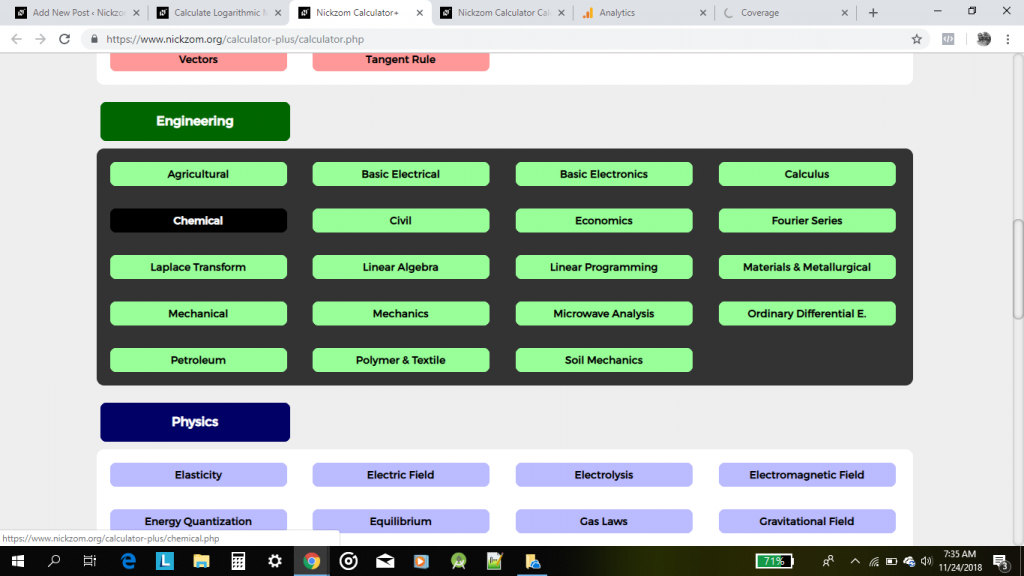Clicking on Chemical under Engineering, displays 3 major topics that can be handled by Nickzom Calculator in Chemical Engineering which are:

• Fluid Mechanics
• Heat Transfer
• Mass Transfer

The Calculator Encyclopedia Calculates the Position of Turning Point for a Vehicle (Vehicle Dynamics)

Calculating the position of turning point for a vehicle must put into consideration the x co-ordinate and y co-ordinate positions of turning point.

There are various parameters you need to be able to calculate these positions. I would start to with how Nickzom Calculator calculates the position of turning point (x co-ordinate).

You are required to have 4 parameters to get the answer for the position and these parameters are:

• Steer Angle of the Rear Outer Wheel
• Rear Track
• Distance of Rear Wheel from Mass Center
• Steer Angle of Rear Inner Wheel

To access this functionalities in Nickzom Calculator, you need to purchase our professional version – Nickzom Calculator+ (The Calculator Encyclopedia). You can access this software via any of these channels:

With that done, Open up the app, select Engineering and click on Open Section.Click on MechanicalThen, click on Vehicle Dynamics

Nickzom Calculator Calculates Warp Weight in Polymer and Textile Engineering – The Calculator Encyclopedia

According to Wikipedia,

The warp is the set of yarns or other elements stretched in place on a loom before the weft is introduced during the weaving process. It is regarded as the longitudinal set in a finished fabric with two or more sets of elements.

The term is also used for a set of yarns established before the interworking of weft yarns by some other method, such as finger manipulation, yielding wrapped or twined structures. Very simple looms use a spiral warp, in which the warp is made up of a single, very long yarn wound in a spiral pattern around a pair of sticks or beams.

Just like Weft, Warp is a basic component used in weaving to turn thread or yarn into fabric. The longitudinal warp yarns are held stationary in tension on a frame or loom. A pick is a single thread of the weft crossing the warp. Each individual warp thread in a fabric is called a warp end or end.

I am pleased to inform you that Nickzom Calculator is able to relieve you off the stress of calculating warp weight in kilogram. In a matter of seconds you can find the warp weight with the knowledge of these 3 parameters:

• Total Ends
• Total Length in m
• Warp Count

As always visit the Calculator Map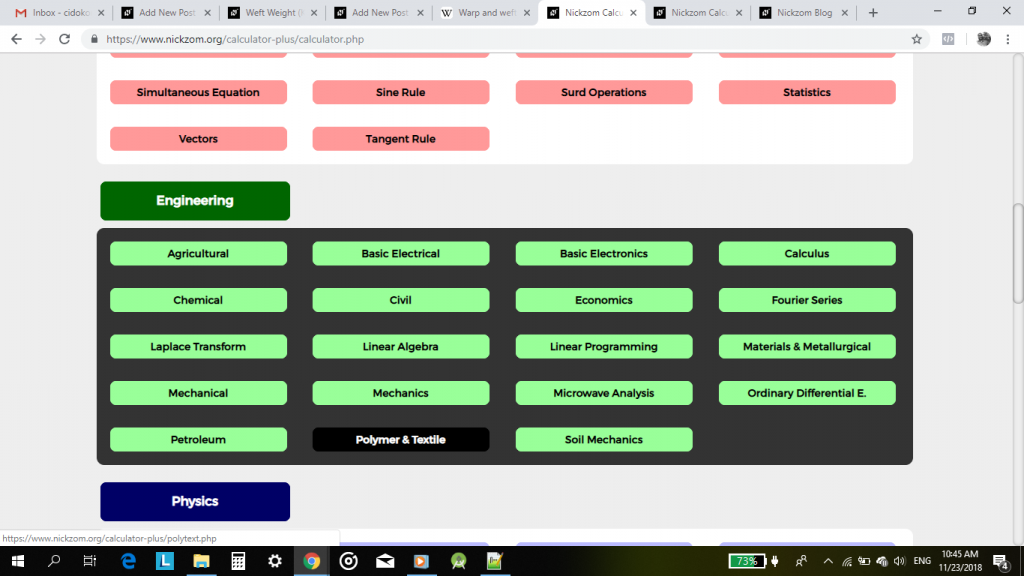Then, scroll down to the engineering section and click on Polymer & Textile

Nickzom Calculator Calculates Weft Weight in Polymer and Textile Engineering – The Calculator Encyclopedia

Weft is a basic component used in weaving to turn yard or loom into fabric.  The transverse weft is drawn through and inserted over and under the warp. A single thread crossing the warp is called a pick.

According to Wikipedia,

Because the weft does not have to be stretched on a loom the way the warp is it can generally be less strong. It is usually made of spun fibre, originally woolflax and cotton, today often of synthetic fiber such as nylon or rayon.

The weft is threaded through the warp using a “shuttle“, air jets or “rapier grippers”. Hand looms were the original weaver’s tool, with the shuttle being threaded through alternately raised warps by hand.

Nickzom Calculator calculates a bunch of calculations in Polymer and Textile Engineering and shows the steps (workings), formulas and answers. Today, I would showing you with pictures how The Calculator Encyclopedia can calculate the Weft Weight in Kilograms (Kg).

First, you need to have access to the professional version of Nickzom Calculator (Nickzom Calculator+) via any of these channels:

Select Engineering and Click on Open SectionA list of Engineering Majors of which its calculations can be handled by the calculator encyclopedia is listed out in alphabetical order. Scroll down and click on Polymer & Textile.The Polymer and Textile Engineering Page has over 200 calculative parameters. Scroll down to Weft Weight (Kg) and click on it.

The Calculator Encyclopedia Calculates the Length of An Open Belt Drive in the Transmission of Power by Belts and Ropes

Calculating the length of an open belt drive is a common thing in Mechanical Engineering. It was of those crucial parameters in the determining the transmission of power by belts and ropes.

In our world today, there are a lot of mechanical engines making use of belts and I believe as a Mechanical Engineer having access to Nickzom Calculator+ is one of the best tools to make lifer easier, faster and simpler.

Nickzom Calculator+ (Professional Version) – The Calculator Encyclopedia can solve over 10,000 calculations for a day to day Mechanical Engineer and today I would show you the steps on how to get the answer for the length of an open belt drive using Nickzom Calculator.

First and Foremost, you need to have access to Nickzom Calculator+ via any of these means:

You must also know that to get the length of an open belt drive there are 3 major parameters you need which are:

• Distance between the Centres of the Larger and Smaller Pullerys
• Radius of the Larger Pulley
• Radius of the Smaller Pulley

Once, you have access to the professional version of Nickzom Calculator via any means, move over to the calculator map, then scroll to the Engineering section and click on Mechanical.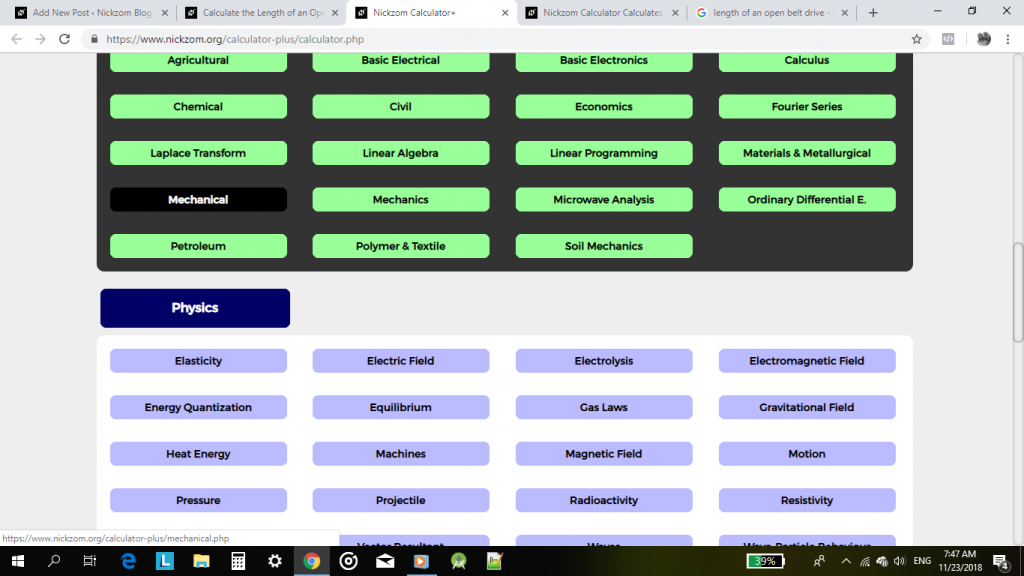On clicking on Mechanical, you see a bunch of major topics that can be solved by Nickzom Calculator. Click on Transmission of Power by Belts and Ropes.